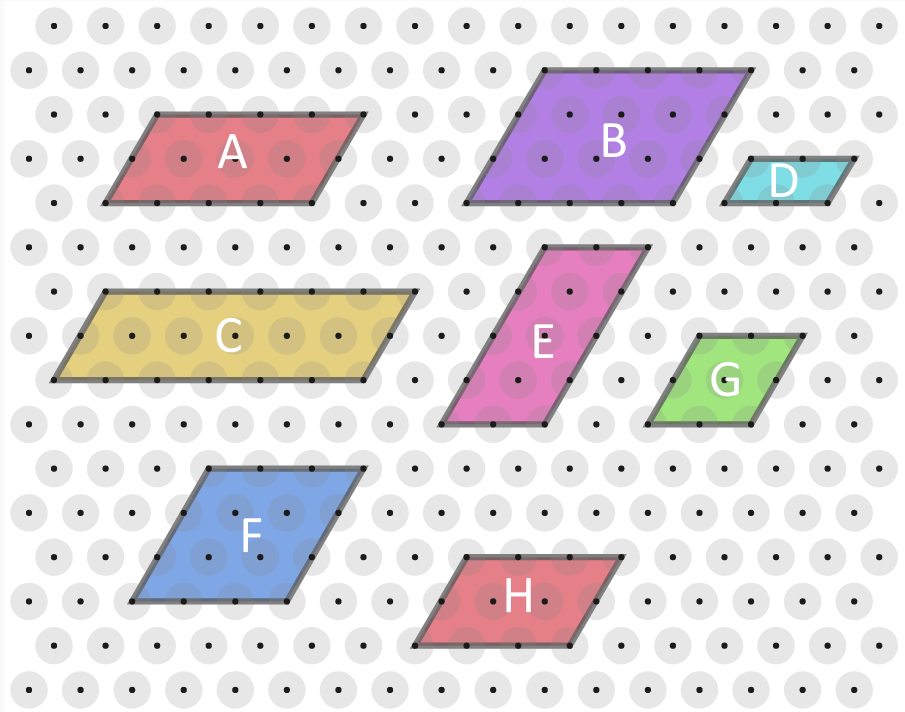#### You may also like### Kissing Triangles

Determine the total shaded area of the 'kissing triangles'.### Isosceles

Prove that a triangle with sides of length 5, 5 and 6 has the same area as a triangle with sides of length 5, 5 and 8. Find other pairs of non-congruent isosceles triangles which have equal areas.Four rods, two of length a and two of length b, are linked to form a kite. The linkage is moveable so that the angles change. What is the maximum area of the kite?

# Isometric Areas

##### Age 11 to 14Challenge Level

You may wish to print off some isometric paper.

Here is an equilateral triangle with sides of length 1.Let's define a unit of area, $T$, such that the triangle has area $1T$.

Here are some parallelograms whose side lengths are whole numbers.Can you find the area, in terms of $T$, of each parallelogram?
Compare the results with the lengths of their edges.

What do you notice?
Can you explain what you've noticed?

Can you find a similar result for trapeziums in which all four lengths are whole numbers?

You might like to try More Isometric Areas next.